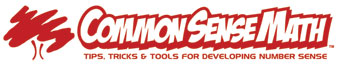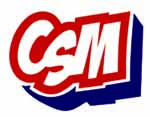Home | FAQs | Contact Us | School Login
 Home About Us How itWorks StandardsAlignment ResearchedStrategies GradeLevels ClassroomTeacher Kits UpcomingWorkshops FAQs Pricing &Ordering

# CSM works within Common Core State Standards (CCSS)

CCSS - Learning Progressions by Domains & Grade Levels

 Kdg 1 2 3 4 5 6 7 8 HS Counting & Cardinality Number and Quantity Number and Operations in Base Ten The Number System Number & Operations Fractions Ratio & Proportional Relationships Operations & Algebraic Thinking Expressions and Equations Algebra Functions Functions Geometry Geometry Geometry Measurement & Data Statistics & Probability Statistics and Probability

 "CCSS - Content Standards" CCSS Content Standards are listed by Domains (or general concepts). Notice the progression from elementary to middle school to high school. Below are general descriptions of Domains for Kdg through 8th Grade. Remember that each grade level will have its own appropriate expectations and build on each other towards High School. CSM Workouts fit within the CCSS Domains. "CCSS - Practice Standards" CSM has always been designed to get students thinking, talking and proving math patterns and concepts. With repetition, students will be able to discover and reason for themselves the patterns and "short-cuts" they find. CSM helps develop a strong, permanent "number sense", so students have the tools they need at each level. 1) Make sense of problems & persevere in solving them (Get the main idea of what is being asked & what is known before starting to solve. Continually ask "is this making sense?" throughout the solution process.2) Reason abstractly & quantitatively (make sense of numbers and quantities and connect their relationships3) Construct viable arguments & critique the reasoning of others (use math concepts and previous results to make a logical progression to the conclusion. Defend it with facts)4) Model with mathematics (use models, graphic organizers, graphs, charts and math "sentences" or expressions & equations to represent a situation. Include mental and visual models) 5) Use appropriate tools, strategically (many technology & tools are available, including mental, visual, & modeling - know when to use what, appropriately)6) Attend to precision (work accurately and communicate with others using details)7) Look for and make use of structure (find, analyze and prove patterns, then apply them)8) Look for and express regularity in repeated reasoning (look for both general methods and short-cuts, evaluate reasonableness)Basic Computation is "The Trunk of the Tree" that Feeds all Standards If students are relying on crutches for basic facts (fingers, charts, calculators) it affects all the other Standards. Students become frustrated and new concepts are difficult to grasp without common math vocabulary and good computation skills. These skills are the "trunk of the tree" that feeds all the other Standards. Review Previous Skills & Build On Them Effective instructional strategies say to review and activate previous skills (bring things to the front of student's minds) before adding to & building upon that skill. Doing a Workout over a previously tested skill, helps students grasp the new skill being introduced even if it is not a "testable objective" for that grade.Parents also have a quick & easy way to refresh skills they haven't used in a while. Customize to Specific Needs of Students CSM Workouts are generally aligned to fit within all Standards, as needed. Common Sense Math is a "tool" that gives a tremendous amount of flexibility & creativity to pull needed skills into any curriculum. The ability to customize & differentiate lessons according to the needs of your students is powerful. For example, lowest terms are needed for middle school concepts of Ratios, Proportions and Probability. Your students will benefit from having the ability to "dust off cob webs" and review the previous skill of lowest terms. Now students are able to more easily grasp and build new conceptsCOUNTING & CARDINALITY (KDG) Number names say and write; counting to 100 by 1's and 10's, sequencing by ones (not just from 1); seeing a group of objects and knowing how many (for example, 5 on a die has a spatial pattern that should be recognizable when counting other objects)NUMBERS AND OPERATIONS IN BASE TEN (K-5) & FRACTIONS (3-5) Basic Computation (add, subtract, multiply, divide) with whole numbers, decimals & fractions (3-5); place value; mental math skills & estimation; different representations of numbers (ex .34 = 34/100 = 15/50 = 34%); properties of operations like commutative, associative, distributive and identity. THE NUMBER SYSTEM (6-8) Extend Basic Computation (add, subtract, multiply, divide) with whole numbers, decimals & fractions and include integers (negative numbers); include exponents, square and cube roots; rational and irrational numbersRATIOS, PROPORTIONAL RELATIONSHIPS (6-7) Understand rates and unit rates; use rates and unit rates to solve problems of all kinds.NUMBER & QUANTITY (HS) OPERATIONS & ALGEBRAIC THINKING (K-5) Understanding that math "sentences" (expressions and equations) represent math situations. For example, 3 dice that are all showing 5, can be written as 3 x 5 = ? or 2 times a number plus 1 can be written as 2n + 1 = ?; find & prove patterns; use order of operations, including parenthesis & other grouping symbols to group operations when needed. EXPRESSIONS & EQUATIONS (6-8) extend previous expressions and equations; solve equations; use properties of operations to make equal expressions and equations; independent & dependent variables; linear equations and systems of equations. FUNCTIONS (8) Know that functions have only one output for each input and when graphed a set of ordered pairs is the input and the corresponding output; y = mx + b is a linear function and makes a straight line on a graph.ALGEBRA (HS)FUNCTIONS (HS) GEOMETRY (K-5) Reason with shapes and their characteristics like squares, rectangles, triangles, parallelograms, and rhombus. Understand lines, angles, rays. Coordinate plane and graphing. GEOMETRY (6-8) Extend previous knowledge of shapes and know formulas  include circles, 2-D and 3-D shapes; solve problems with scale; translations & dilations; include surface areaGEOMETRY (HS) MEASUREMENT & DATA (K-5) Classify objects; tell & write time; know money; use rulers & measuring tools; customary & metric; area, perimeter and volume; line plots, bar graphs, picture graphs and more STATISTICS & PROBABILITY (6-8) Know difference of measures of center (mean and median) and measure of variance (range and deviation) and conclude; analyze data in various forms of graphs and graphic representations; Investigate patterns in data; determine outliers; probability of a chance event.STATISTICS & PROBABILITY (HS)